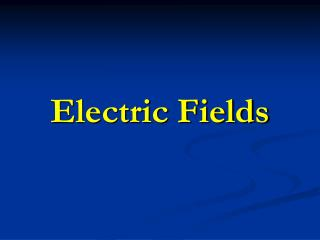DownloadDownload PresentationElectric Fields

# Electric Fields

Download Presentation## Electric Fields

- - - - - - - - - - - - - - - - - - - - - - - - - - - E N D - - - - - - - - - - - - - - - - - - - - - - - - - - -
##### Presentation Transcript

1. Electric Fields

2. F F + What is an electric field??? • It is an area of influence around a charged object. • Positively charged objects within that field will experience an electrical force. - + + - - + - - + + - - - - + + - - + - - + - + + - Test Charge Electric Field Electric Field

3. Calculating the magnitude of an electric field • The magnitude is proportional to the amount of electrical force exerted on the positively charged object. Electric Field Strength = electric force/test charge E =Felectric/q0 SI Unit = N/C

4. Sample Problem Mr. Luna pulls a wool sweater over his head, which charges his body as the sweater rubs against his cotton shirt. What is the electric field strength at a location where a 1.60 x 10-19 C piece of lint experiences a force of 3.2 x 10-9 N as it floats near Mr. Luna? q0 = 1.60 x 10-19 C F = 3.2 x 10-9 N E = Felectric/q0 = 3.2 x 10-9 N / 1.60 x 10-19 C E = 2.0 x 1010 N/C

5. Calculating the strength of an electric field • The strength of an electric field depends on: 1. the charge produced by the electric field 2. the distance between the test charge and the electric field Electric Field Strength = Coulomb constant x field charge/ (distance)² E = (Kc x q)/r² Kc = 8.99 x 10 9 N • m² / C²

6. Sample Problem A fly accumulates 3.0 x 10-10 C of positive charge as it flies through the air. What is the magnitude and direction of the electric field at a location of 2.0 cm away from the fly? q = 3.0 x 10-10 C r = 0.02 m Kc = 8.99 x 109 N • m² / C² E = (Kc x q)/r² =(8.99 x 109 N•m²/C²)(3.0 x 10-10 C) (0.02 m)² E = 6743 N/C away from the fly

7. + - Electric Field Lines • Represent the magnitude and direction of an electric field with respect to the positive test charge. More arrows = greater magnitude

8. Electric Field Lines • When two point charges of equal magnitude, but opposite signs interact with each other, they form an “electric dipole”.

9. Electric Field Lines • Like charged objects that interact with each another, have their field lines moving away from one another.

10. Electric Field Lines • When interacting charges are of a different magnitude, only a fraction of the field lines from the greater charge will move to the lesser charge.